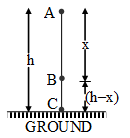# Physics

### Chapter : 3. Work and Energy

#### Inter conversion of potential And kinetic energy

Mechanical Energy of a Freely Falling Body :
Assume, a body of mass m is at rest at a height h from the earth's surface, as it starts falling, its velocity after travelling a distance x (point B) becomes v and its velocity on the earth's surface is v'.
Mechanical energy of the body at point A :
EA = Kinetic energy + Potential energy
EA = m(0)2 + mgh
EA = mgh ………(i)

Mechanical energy of the body at point B :
EB =mv2 + mg (h – x) ……. ..(ii)Mechanical energy of the body at point C :
EC =m (v')2 + mg × 0
EC =m (v')2 ……..(iv)
Use : EA = EB = EC
Hence, when a body falls freely, its mechanical energy will be constant. That means, the total energy of the body during free fall, remains constant at all positions. However, the form of energy keeps on changing at all points during the motion.